# Polynomial ring

In algebra, the polynomial ring over a ring is a construction of a ring which formalises the polynomials of elementary algebra.

## Construction of the polynomial ring

Let R be a ring. Consider the R-module of sequences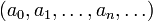$\left(a_0, a_1, \ldots, a_n, \ldots \right) \,$

which have only finitely many non-zero terms, under pointwise addition$(a+b)_n = a_n + b_n .\,$

We define the degree of a non-zero sequence (an) as the the largest integer d such that ad is non-zero.

We define "convolution" of sequences by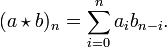$(a \star b)_n = \sum_{i=0}^n a_i b_{n-i} .\,$

Convolution is a commutative, associative operation on sequences which is distributive over addition.

Let X denote the sequence$X = (0,1,0,\ldots) .\,$

We have$X^2 = X \star X = (0,0,1,0,\ldots) \,$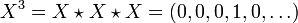$X^3 = X \star X \star X = (0,0,0,1,0,\ldots) \,$

and so on, so that$\left(a_0, a_1, \ldots, a_n, \ldots \right) = \sum_n a_n X^n ,\,$

which makes sense as a finite sum since only finitely many of the an are non-zero.

The ring defined in this way is denoted R[X].

### Alternative points of view

We can view the construction by sequences from various points of view

We may consider the set of sequences described above as the set of R-valued functions on the set N of natural numbers (including zero) and defining the support of a function to be the set of arguments where it is non-zero. We then restrict to functions of finite support under pointwise addition and convolution.

We may further consider N to be the free monoid on one generator. The functions of finite support on a monoid M form the monoid ring R[M].

## Properties

• If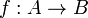$f:A \rarr B$ is a ring homomorphism then there is a homomorphism, also denoted by f, from$A[X] \rarr B[X]$ which extends f. Any homomorphism on A[X] is determined by its restriction to A and its value at X.

## Multiple variables

The polynomial ring construction may be iterated to define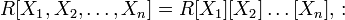$R[X_1,X_2,\ldots,X_n] = R[X_1][X_2]\ldots[X_n] ,\,:$

but a more general construction which allows the construction of polynomials in any set of variables$\{ X_\lambda : \lambda \in \Lambda \}$ is to follow the initial construction by taking S to be the Cartesian power$\mathbf{N}^\Lambda$ and then to consider the R-valued functions on S with finite support.

We see that there are natural isomorphisms$R[X_1][X_2] \equiv R[X_1,X_2] \equiv R[X_2][X_1] .\,$

We may also view this construction as taking the free monoid S on the set Λ and then forming the monoid ring R[S].